Top Menu Next Previous Articles Paleo-Horsemen Nasca Monkey

The Seal of Atlantis

The Cone & Square formation :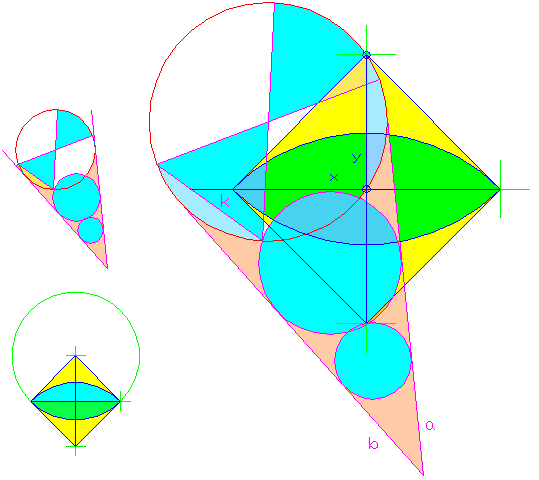The mystery of the Cone The K-circle takes part in the balanced layout of Athena from head to toe, as we just saw. Here, the K-circle forms the Cone with two more circles . 1) The centers of the K-circle and of the two new circles lay on one line. 2) In addition, the three circles are symmetrical (have common external tangents).  The external tangents, which demonstrate that the three circles are symmetrical, and the circles form the Cone.             The Cone's middle circle Unlike the K-circle, the other two Cone circles reappear throughout the engraving. For instance, the arcs on Athena's right leg (which we saw imply an equilateral triangle) have the same radius as the Cone's  middle circle.  How can I say so? After all, due to circumstances, when a given arc indicates a circle,  that circle is not sharply defined, but rather it has a narrow range of possibilities, which all look just about as good, as they all seem to follow the arc. How do we determine, what circle best fits a given arc?  Such a circle should fit nicely not only the arc, but also the picture:  Is the circle's center signalled by a marked point in the engraving?  Or, does it sit on a line's edge? Is the circle propped against other lines, and line ends?  Does it consistently pass through points like line crossings? Does it seem to be of the same size as a group of other circles? _If so, this should be the right circle, because it looks deliberate in all aspects. So, there I was - crazy enough to draw regular geometrical figures over a 14,000 years old engraving. I had the Square, and the Cone, and the fact that some circles were reiterated in a number of places.  Were they standard?                                                      *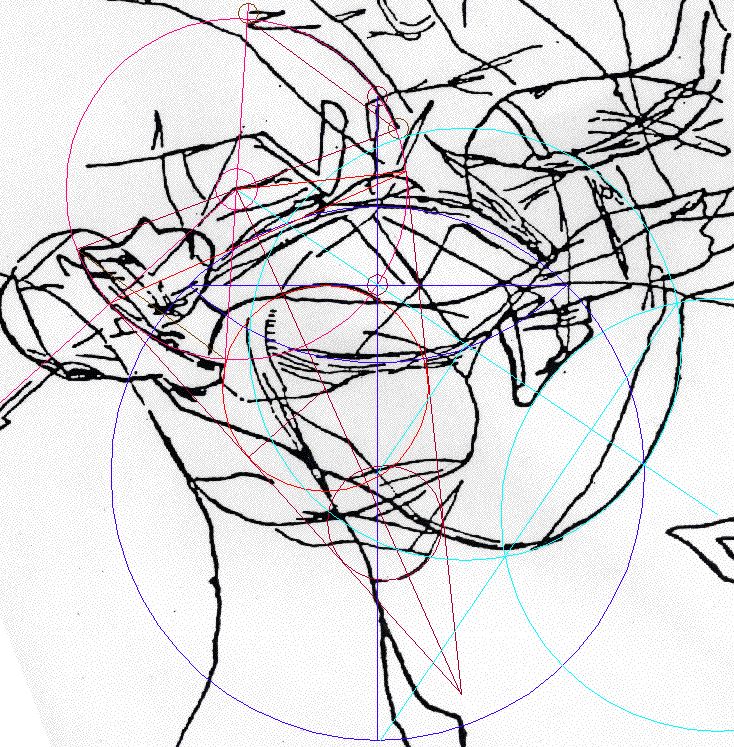The Cone cannot be solved without the next clue:    The Cone duplicates the angle at the tip of a 5-pointed star.     So, was the Cone drawn from a 5-pointed star?  At this stage, I started to seriously search for a 5-pointed star, which would explain the specifics of this Cone.

The Big Breakthrough

Quite a few arcs in the engraving of Athena translate into circles just like the Cone's middle circle.
Based on this frequency of appearance, the Cone's middle circle could conceivably be a unit circle. Therefore, measuring the Cone with it is natural, and that's what I did.

(diag.below).
step by step procedure:
I drew five unit circles spaced by the radius along each arm of the Cone. Then I drew the same circle from the center of the K-circle. It was a perfect tangent to the two nearest unit circles on the Cone, thus capping the Cone.

Cone Mapping.diag

 Top Menu Abydos Helicopter Prehistoric message in thirteen numbers Articles Paleo-Horsemen Nasca Monkey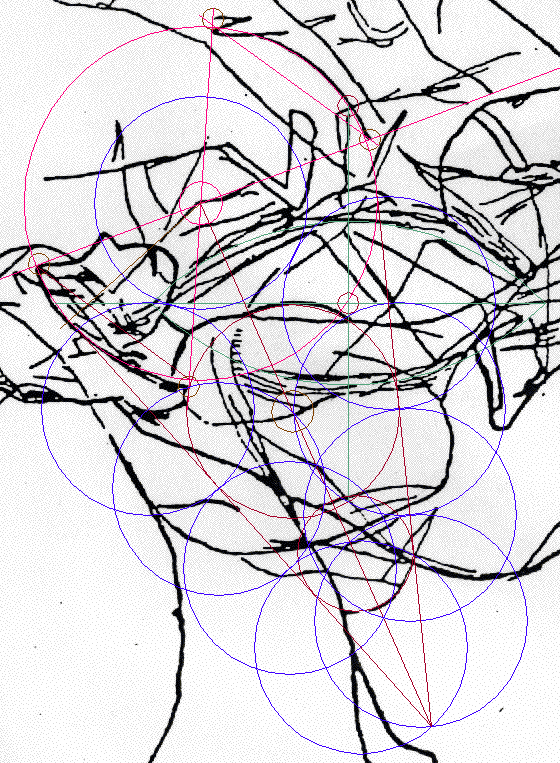Since measuring out 5 unit-circle radii along the Cone's sides is is the key part to its solution, does this indicate that the hypothetical star to be imposed upon the 36 degree Cone should have arms 5 unit-unit circle radii long?Problems of perception The new Cone-star (S-star) creates some special visual effects. Drawing the circumcircle of the Cone-star concentrically with one of the Torso circles, shows that the two radii differ by one pixel only. (diag. above) The Cone's circumcircle also seems to be a perfect tangent to one side (north-east) of the Main  Square.  At the time of the experiment, I was quite perplexed by this. Did the construction of the Square from the Cone exactly  duplicate the Cone's circumcircle on the Torso?  Note that when drafting by hand, a  similar question arises with the so called Circle-Triplets, shown in  the diagram below. All three seem close to being identical in size.  But, are they identical? _ My curiosity went unsatisfied until I could recreate the position in CAD, on a \$2,000, 10 MHz pc :)    The Circle-Triplets Take a circle, which passes through the inside  points (corners) of our 5-pointed star.This circle (dubbed Inner-circle) on our experimental Cone-star seems to be just as big as Unit-circle, but also just as big as the Middle-circle of the Cone. On paper, the radii of both the inner and the middle circles fit the Cone-star's arm a near perfect  five-times, once a hair short - and once a hair too long.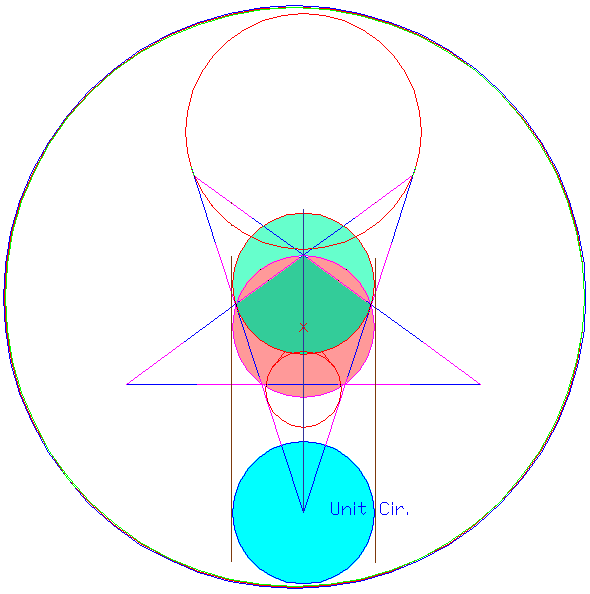In the diagram above, the three CAD produced Triplet circles were made concentric, and then highly magnified. Yet, despite their magnification, these three circles look like a single circle. But, the following is true:                          If the Unit circle's radius  =                       then the Inner circle's radius  =  [1.0040..]                         radius of the Middle-circle = [0.9975..] One mathematical consequence of this unit system: The side of a pentagon inscribed into the 'inner'-circle measures         1.1180339887... -   the square root of 5 divided by 2 The PHI-ratio equals the same square root of  5 divided by 2   +  0.5                1.11 80339887... + 0.5  =  1.6180339887.. Now, measuring of the Cone by the unit-circles makes perfectly good sense.The unit circles describe the Cone perfectly. The experiment was a big success. Now, when we view the the Cone, as it is logically described by the unit circles, we actually see its geometrical solution, as well. And, when we review  this solution together with the Square, we realize that this solution solves its origin (construction) too! Reverse of the Cone mapping procedure We used the Cone for the mapping process. The logic behind the results of the mapping makes it possible to reverse the process. Starting from scratch, we can reproduce the mapped cone, and then use it to get an exact facsimile of the original Cone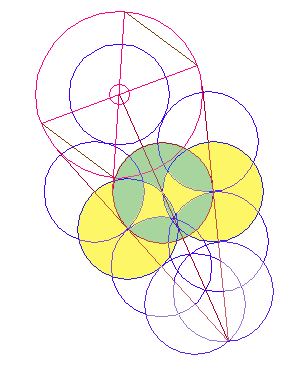The size and position of the K-circle on the Cone The K-circle was generated from the capping circle's center as a tangent to the Cone's sides. But, this center-point may be found by  an even quicker method - the upper intersection of the third row of  the mapping circles on the central axis marks the mid-point between the K-circle's center, and the tip of the Cone.  The size and position of the Middle-circle on the Cone It is also derived from the unit circles describing the Cone: the fourth row of  these circles (whose centers are 3 radii from the tip of the Cone) intersects at the center of the Middle circle (diag. below).The size and position of the Small-circle on the Cone It is meant to fit between:                                           a) the internal tangents                                          b) the external tangents (Cone's sides).                                                     *  It is logical to reason that the Cone and its circles are derived from a regular 5-pointed star (the Mother Star).  The size and position of the Square with respect to the Cone The self-explanatory Cone also explains the Square's construction. The Square -  derives from the Cone in a process requiring just three circles: the K-circle, and two of the mapping Unit-circles. Procedure: The Square's horizontal diagonal rests upon two of the unit circles.<>The K-circle intersects the center of the Square on the horizontal  diagonal, which we already have. From there, we then draw the vertical diagonal of the Square. The higher point, at which the K-circle crosses the vertical diagonal,  marks the Square's top corner.

 The Cone, the Mother-star & Geometry The Cone's concept turns out utterly methodical, and scientific. Neither Euclid nor Pyrthagoras could improve upon it. Our  caveman thinks, as if he were a professor of mathematics.  I had come across some diagrams looking like the Cone, in a collegiate handbook on geometry. The diagrams dealt with the topic of similarity of  circles: That's when I learned that theory calls the Cone's sides "common  external tangents - and the Cone's tip the "external center of similitude. Next, the book revealed that there are internal counterparts to these: 1) the Internal Tangents between the Cone's top, and small circles. 2) the Internal Center of Similitude, at the point S.Click for animated construction of the tangents (26 Kb) In the illustration above, we use parallel diameters of two circles to find their centers of similitude, or symmetry: A line between opposed ends of the diameters intersects the line of circle-centers at the internal center of symmetry "S" _  An internal tangent is drawn from S to both circles. A line between the correspondent ends of the diameters intersects the line of circle-centers at the external center of symmetry "V". An external tangent is drawn from V. The Ancients had indicated their knowledge of external tangent construction by symbolizing the Cone. But, could they have indicated their knowledge of the complementary internal tangent construction? Well, our own construction of internal tangents between the Cone's top and bottom circles results in a spectacular effect..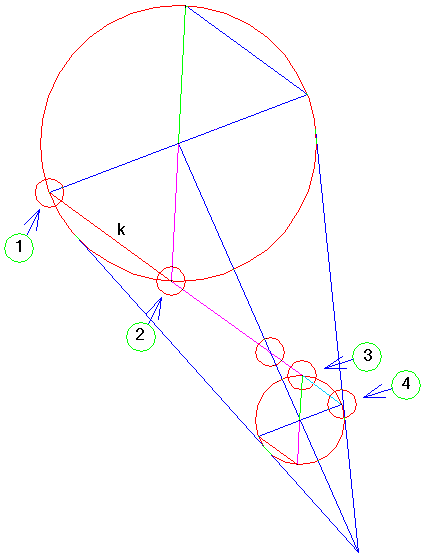A Rare Illusion - Six lines fuse to look like a single line In this situation, the four numbered points produce six different lines.  However, all these straight lines give an illusion of being one and the same line (see diag. above). The effect is as beautiful, as it is unlikely. The indication is that the Ancients knew, and played with, the idea of internal tangents. Now, the Cone's external center of symmetry sets one tip of its star. To be consistent,  the Ancients should have used the Cone's internal center  of symmetry for the center of its 5-pointed star. - This would explain  the smallest circle of the three on the Cone, as wedged between the  internal and external tangents. The internal tangent was first drawn as a tangent to the K-circle from the 5-pointed star's center, then it was extended, and then the small circle was drawn.   Go to animation on the Triplets

© Jiri Mruzek  (to write me, use Yahoo.com +  my name without the space)                    Discussion Forum
You can use a fake e-mail to log in

 Top Next Previous Stone Age Horsemen Articles Nasca Monkey